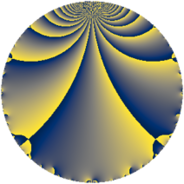# Properties

 Label 34.2.dLevel $34$ Weight $2$ Character orbit 34.d Rep. character $\chi_{34}(9,\cdot)$ Character field $\Q(\zeta_{8})$ Dimension $4$ Newform subspaces $1$ Sturm bound $9$ Trace bound $0$

# Related objects

## Defining parameters

 Level: $$N$$ $$=$$ $$34 = 2 \cdot 17$$ Weight: $$k$$ $$=$$ $$2$$ Character orbit: $$[\chi]$$ $$=$$ 34.d (of order $$8$$ and degree $$4$$) Character conductor: $$\operatorname{cond}(\chi)$$ $$=$$ $$17$$ Character field: $$\Q(\zeta_{8})$$ Newform subspaces: $$1$$ Sturm bound: $$9$$ Trace bound: $$0$$

## Dimensions

The following table gives the dimensions of various subspaces of $$M_{2}(34, [\chi])$$.

Total New Old
Modular forms 28 4 24
Cusp forms 12 4 8
Eisenstein series 16 0 16

## Trace form

 $$4 q - 8 q^{5} - 4 q^{9} + O(q^{10})$$ $$4 q - 8 q^{5} - 4 q^{9} - 8 q^{10} + 4 q^{11} + 4 q^{12} + 8 q^{14} + 8 q^{15} - 4 q^{16} + 4 q^{18} + 8 q^{19} + 8 q^{22} - 16 q^{23} + 4 q^{24} + 16 q^{25} - 8 q^{26} - 12 q^{27} - 8 q^{28} - 16 q^{33} - 16 q^{34} - 4 q^{36} - 8 q^{37} + 16 q^{41} + 8 q^{42} + 12 q^{43} + 8 q^{44} + 8 q^{45} - 16 q^{49} + 12 q^{50} + 16 q^{51} + 8 q^{52} + 8 q^{53} + 4 q^{54} + 8 q^{57} - 4 q^{59} - 8 q^{60} + 16 q^{61} - 8 q^{63} - 16 q^{65} - 12 q^{66} - 24 q^{67} - 16 q^{70} - 8 q^{71} + 8 q^{74} - 28 q^{75} - 8 q^{76} + 8 q^{77} - 8 q^{79} + 8 q^{80} + 20 q^{82} + 12 q^{83} - 8 q^{85} - 24 q^{86} + 8 q^{87} + 4 q^{88} + 32 q^{91} - 8 q^{93} + 16 q^{94} - 12 q^{97} + O(q^{100})$$

## Decomposition of $$S_{2}^{\mathrm{new}}(34, [\chi])$$ into newform subspaces

Label Dim $A$ Field CM Traces $q$-expansion
$a_{2}$ $a_{3}$ $a_{5}$ $a_{7}$
34.2.d.a $4$ $0.271$ $$\Q(\zeta_{8})$$ None $$0$$ $$0$$ $$-8$$ $$0$$ $$q+\zeta_{8}^{3}q^{2}+(\zeta_{8}^{2}-\zeta_{8}^{3})q^{3}-\zeta_{8}^{2}q^{4}+\cdots$$

## Decomposition of $$S_{2}^{\mathrm{old}}(34, [\chi])$$ into lower level spaces

$$S_{2}^{\mathrm{old}}(34, [\chi]) \cong$$ $$S_{2}^{\mathrm{new}}(17, [\chi])$$$$^{\oplus 2}$$